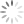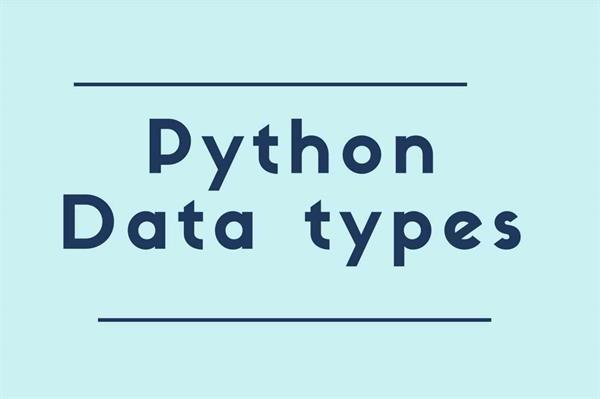Loading, please wait...# Data Types in PYTHON

Jul 18, 2020 Data Types , 2426 Views

Through this article, we will learn about Built-in data types in python. In each programming language data type is one of the important concepts.

In python programming, we don’t need to declare the type of any variable as we know python is a dynamical type ( i.e unlike other programming languages there is no strict declaration before assigning values to them).

The type of variable is defined at runtime by the python interpreter. If you want to understand the variable in python, then I would suggest following my previous article “Variable in Python”. Through that article, the concept of the variable will be clear for you.

Data typing helps the processor to allocate the memory space for the variable according to their type. As we know python is an object-oriented programming language so each and everything in python is an object.

Data type means a type of a variable or we can say that data type is a class and variable is an instance of an object of the class Data type.The built-in data types in python are listed in the table.

• Text type: String
• Sequence type: List, Tuples, Range
• Mapping type: Dictionary
• Boolean type: Bool
• Binary type: Bytes, Byte Array
• Set Type: Set
• Numeric Type: Integer, Float, Complex

Type() Function:

In python, type function is used to get the data type of the variable. As we know the value assigned to variable defines the data type of that variable.

Code:

``````name = 'Tutorial Link'
print(type(name))
#Output:<class 'str'>``````

In python, the data type of a variable is set when we assign a value to a variable.

Code:

``````#Text type: string
print(type(name))
#output:<class 'str'>

#Sequence type
#List:
name=["Rohan" , "Mohan" , "Sohan"]
print(type(name))
#output:<class 'list'>

#Tuple:
x= ("Riya" , "Priya" ,1.8 ,89)
print(type(x))
#output:<class 'tuple'>

#Range:
y=range(20)
print(y)
#output:range(0, 20)

#Mapping Type : Dictionary
marks={
"English" : 90,
"Hindi" : 90,
"Maths" : 78,
"Science" : 90
}
print(type(marks))
#output:<class 'dict'>

#Boolean Type: Bool
counter = True
order = False
print(type(counter))
print(type(order))
#output: <class 'bool'>
#<class 'bool'>

#Set type: Set
command={ 'remove', 'mkdir' , 'cd' ,'clear' }
var1={ }     #thats dictionary not empty set
var2=set()  #Empty set
print(type(command))
#output:<class 'set'>
print(type(var2))
#output:<class 'set'>
print(type(var1))
#output:<class 'dict'>

#Numeric type:
#Integers
marks = 90
print(type(marks))
#output:<class 'int'>

#Floats
marks=78.5
print(type(marks))
#output:<class 'float'>

#Complex
abc = 5+2j
print(type(abc))
#output:
#<class 'complex'>

#Binary Type:
#Byte
y=b"Hello World"
print(type(y))
#output:
#<class 'bytes'>

#Bytearray
name= bytearray(789)
print(type(name))
#output:
#<class 'bytearray'>
``````

We can also specify the data type using the constructor function. let's discuss through a simple code example.

Code:

``````name = str('Tutorial Link')
name = list(("Rohan" , "Mohan" , "Sohan"))
name = tuple(("Rohan" , "Mohan" , "Sohan"))
name = set(("Rohan" , "Mohan" , "Sohan"))
name = frozenset(("Rohan" , "Mohan" , "Sohan"))
x = int(6)
x = float(9.0)
x = complex(1j)
x = range(8)
x = bool(6)
x = bytes(6)
x = bytearray(6)
marks = dict(subject="Maths" , number=89)
``````